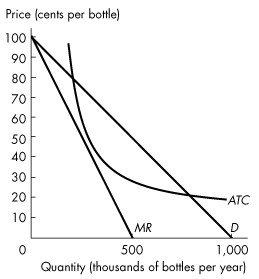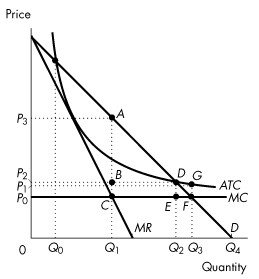/
/
/
13.5   Monopoly Regulation 1) Rate of return regulation can end up
Not my Question
Flag Content

# Question : 13.5   Monopoly Regulation 1) Rate of return regulation can end up : 1418013

13.5   Monopoly Regulation

1) Rate of return regulation can end up serving the self-interest of the firm if

A) there is a great demand for the good.

B) there is a competitive struggle to determine which firms will supply the market.

C) the regulated firm overstates its costs of production.

D) price is set at average total cost.

E) the rate is set too low.

2) A monopolist under rate of return regulation has an incentive to

A) pad costs.

B) produce more than the efficient quantity of output.

C) charge a price equal to marginal cost.

D) maximize consumer surplus.

E) maximize shareholder profits

3) A monopolist under marginal cost pricing has an incentive to

A) inflate costs.

B) produce more than the efficient quantity of output.

C) produce less than the efficient quantity of output.

D) maximize consumer surplus.

E) produce the efficient quantity of output.

Use the information below to answer the following questions.

Fact 13.5.1Figure 13.5.1

4) Refer to Figure 13.5.1. Suppose the industry is unregulated. In this case, output is

A) 400,000 bottles per year.

B) 450,000 bottles per year.

C) 600,000 bottles per year.

D) 700,000 bottles per year.

E) 800,000 bottles per year.

5) Refer to Figure 13.5.1. Suppose the firm is regulated by the government that imposes marginal cost pricing. The price of a bottle of water is

A) \$0.

B) \$0.50.

C) \$0.30.

D) \$0.10.

E) \$0.20.

6) Refer to Figure 13.5.1. Suppose the government regulates the firm with average cost pricing. What is the price?

A) \$0 a bottle

B) \$0.50 a bottle

C) \$0.30 a bottle

D) \$0.10 a bottle

E) \$0.20 a bottle

Use the figure below to answer the following questions.Figure 13.5.2

7) Consider the natural monopoly depicted in Figure 13.5.2. If a regulator uses a marginal cost pricing rule to set price, what is the quantity produced?

A) 0

B) Q0

C) Q1

D) Q2

E) Q3

8) Consider the natural monopoly depicted in Figure 13.5.2. If a regulatory agency sets a price just sufficient for the firm to make zero economic profit, what output will it produce?

A) Q4

B) Q0

C) Q1

D) Q2

E) Q3

9) Consider the natural monopoly depicted in Figure 13.5.2. If a regulatory agency sets a price just sufficient for the firm to make zero economic profit, and if the firm inflates its costs as much as possible, the regulated price will be

A) P0.

B) P1.

C) P2.

D) P3.

E) none of the above.

10) Consider the natural monopoly depicted in Figure 13.5.2. What area in the graph represents the deadweight loss arising from an average cost pricing rule?

A) ABD

B) DGF

C) DEF

D) ACF

E) CBDE

## Solution 5 (1 Ratings )

Solved
Economics 1 Month Ago 45 Views
This Question has Been Answered!